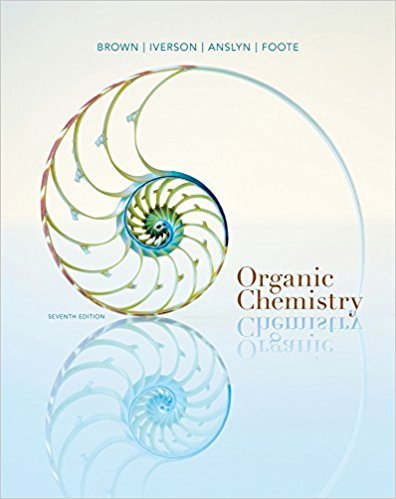×
Get Full Access to Organic Chemistry - 7 Edition - Chapter 15 - Problem 15.25
Get Full Access to Organic Chemistry - 7 Edition - Chapter 15 - Problem 15.25

×

# The synthesis of carbohydrates can be particularly difficult because of the largeISBN: 9781133952848 483

## Solution for problem 15.25 Chapter 15

Organic Chemistry | 7th Edition

• Textbook Solutions
• 2901 Step-by-step solutions solved by professors and subject experts
• Get 24/7 help from StudySoup virtual teaching assistantsOrganic Chemistry | 7th Edition

4 5 1 242 Reviews
23
0
Problem 15.25

The synthesis of carbohydrates can be particularly difficult because of the large numberof chiral centers and OH functional groups present. Epoxides can be useful syntheticintermediates in carbohydrate syntheses. Draw the product of the following reactions ofa Gilman reagent with each epoxide.

Step-by-Step Solution:
Step 1 of 3

Gen Chem Chap 19: Ionic Equilibria in Aqueous System What is a buffer solution Two­compound solutes that resist change in pH when acid or base are added. They maintain a narrow pH range. How many minimum components must an effective buffer contain Two: one for H+, other for OH­ What are the two main types of buffers Weak acid + its conjugate base Weak base + its conjugate acid Write two balance net equations showing how a buffer composed of nitrous acid and potassium nitrite works HNO2 + OH­ = H2O + NO2­ NO2­ + H+ = HNO2 Explain why a solution of sodium chloride and hydrochloric acid is not a buffer solution HCl is a strong acid, buffers require weeklies Explain why a solution formed by mixing 100 ml of a 0.100 M acetic acid with 50 ml of 0.100 M sodium hydroxide solution will act as a buffer solution HAc + OH­ = Ac­ +H2O Given concentrations results in 50% HAc and 50% Ac­ balanced buffer system Write the Handerson­Hasselbalch equation for a weak acid/conjugate base buffer system PH =pKa + log [Conj base/weak acid] Write the Handerson­Hasselbalch equation for a weak base/ conjugate acid buffer system POH= pKb + log [conj acid/weak base] What law was the Henderson­Hasselbalch equation derived from Ans: Law of mass action What is the principle application for the Henderson­Hasselbalch Equation Buffer Solutions Equivalence point Theoretical point during titration when reaction reaches stoichiometric completion End Point Experimental approximation of equivalence point when indicator changes color Titration Caref

Step 2 of 3

Step 3 of 3

##### ISBN: 9781133952848

Unlock Textbook Solution